# MAXIFS Function in Excel

Get the maximum value based on specified criteria

## What is the MAXIFS Function?

The MAXIFS Function in Excel is a Statistical function that returns the maximum value based on one or more than one conditions from the specified cells. The MAXIFS function was introduced in MS Excel 2016.

### Formula

=MAXIFS(max_range, criteria_range1, criteria1, [criteria_range2, criteria2], …)

Arguments:

1. Max_range (required argument) – The actual range of cells from which the maximum value will be determined.
2. Criteria_range (required argument) – The set of cells to be evaluated with the criteria.
3. Criteria1 (required argument) – Here, we give a number, expression, or text that defines which cells will be evaluated as maximum.
4. Criteria_range2 – An optional argument wherein we can specify additional ranges and their associated criteria.

MS Excel allows putting in up to 126 criteria.

### How to use the MAXIFS Function in Excel?

It is a built-in function that can be used as a worksheet function in Excel. Let’s consider a few examples:

##### Example 1

Suppose we are given the quarterly expenses from the three regions in which our business operates. We wish to find out the maximum expense for the North region, so let’s see the formula to be used:

=MAXIFS( \$D\$5:\$D\$33, B\$5:B\$33, “North”)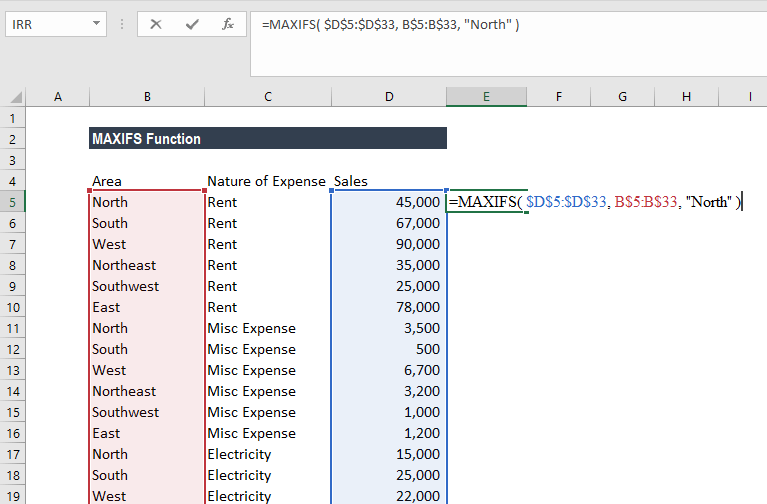In the example above, the Excel MAXIFS function identifies rows where the value in column B is equal to North, and returns the maximum value from the corresponding values in column D.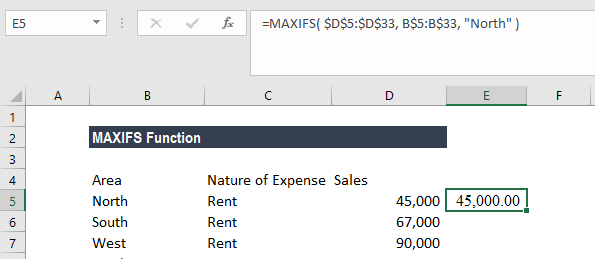In this example, the MAXIFS Function identifies rows where the value in column A is equal to North and returns the maximum value from the corresponding values in column C.

##### Example 2

Let’s continue with the same example. We wish to know which region registered the highest printing and stationary expense. The formula to use will be:

=MAXIFS( \$D\$5:\$D\$33, C\$5:C\$33, “Printing & Stationary” )

The formula will return the amount with the expense of Printing & stationary.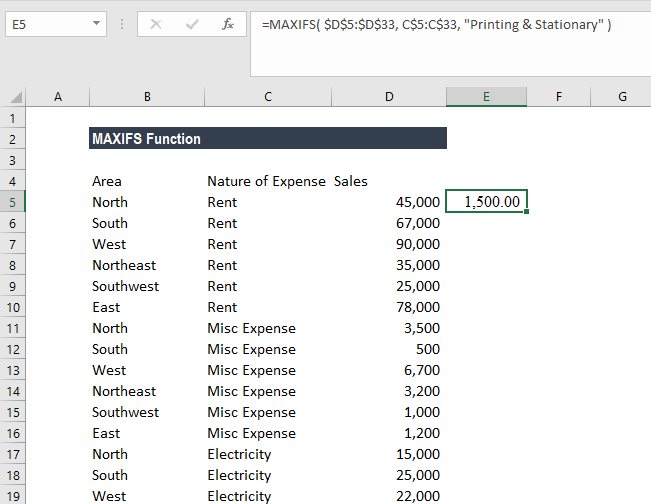##### Example 3

Let’s take another example. We wish to find out the student who scored Distinction in any subject and the marks scored are maximum. We use the data below: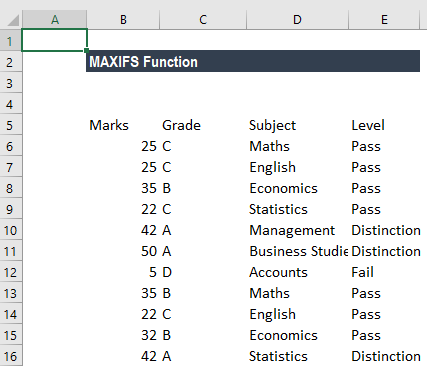The formula to use will be =MAXIFS(B6:B16,C6:C16,”A”,E6:E16,”=Distinction”)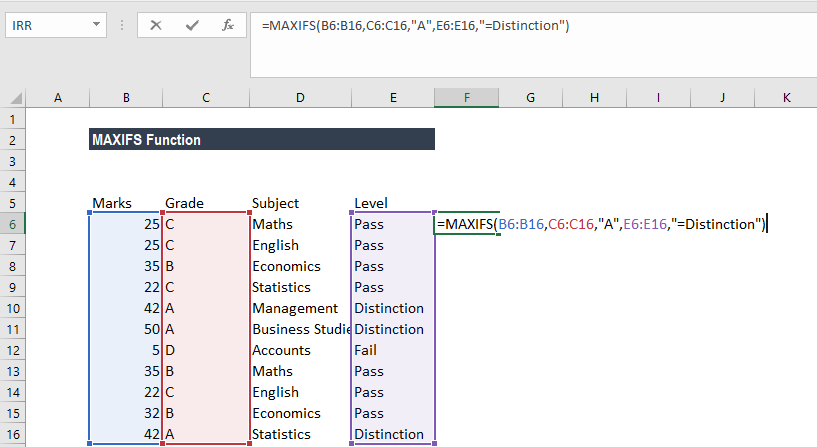In criteria_range1, C10, C11, and C16 match the criteria of “A” of the corresponding cells in criteria_range2, E10, E11 and E16 match the criteria of Distinction. Finally, of the corresponding cells in max_range, B11 gives the maximum value. The result is therefore 50.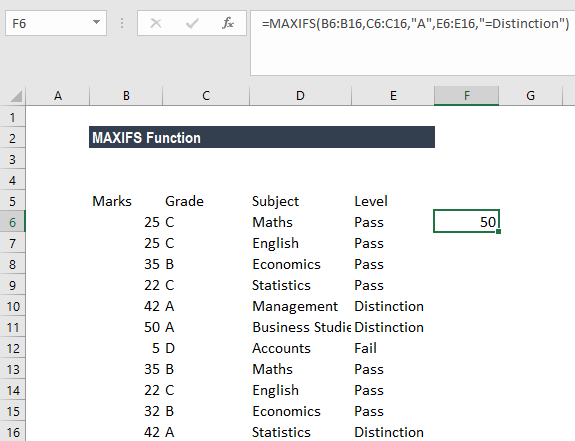##### Example 4

Let’s take this example wherein we would use MAXIFS with an array formula. We wish to use the “MAXIFS” formula that will return the latest date for open tasks for each project in Column E for the data below: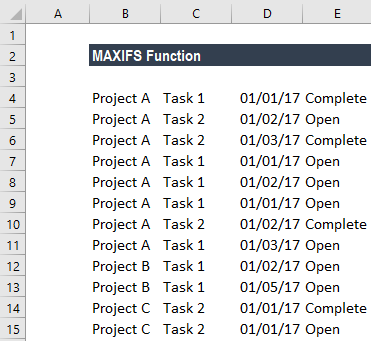So, for the data example above, we would expect to see 01/03/2017 in Column F on all rows for Project A; 01/05/2017 for all rows of Project B; and 01/01/2017 for all rows of Project C.

The formula to be used is below: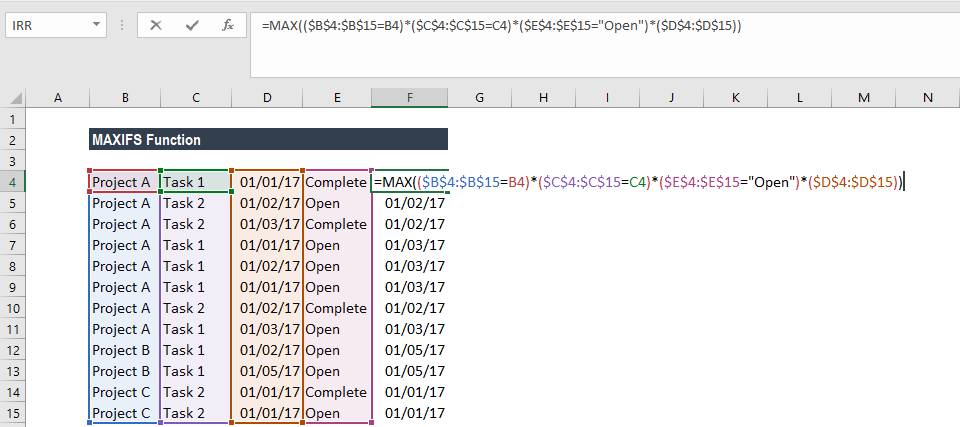For parentheses, we need to press Ctrl+Shift+Enter the first time we input it because it only works as an array formula. After that, we can drag it over the range where we need it.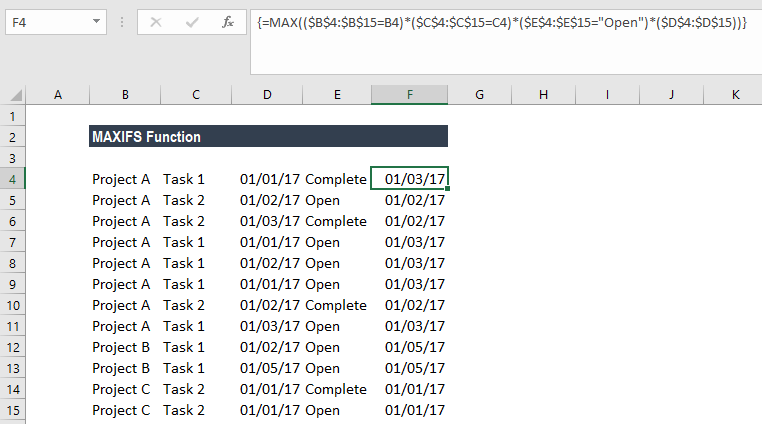### Things to remember about the MAXIFS function

1. #VALUE! error – This is returned when the size and shape of the max_range and criteria_rangeN arguments aren’t the same.
2. If we are using earlier versions of Excel, we can use an array formula based on MAX and IF to find maximum values with criteria.
3. #NAME? error – Occurs when we are using an older function of Excel.
4. MAXIFS will include rows that are hidden.

Thanks for reading CFI’s guide to important Excel functions! By taking the time to learn and master these functions, you’ll significantly speed up your financial analysis. To learn more, check out these additional CFI resources:

• Excel Functions for Finance